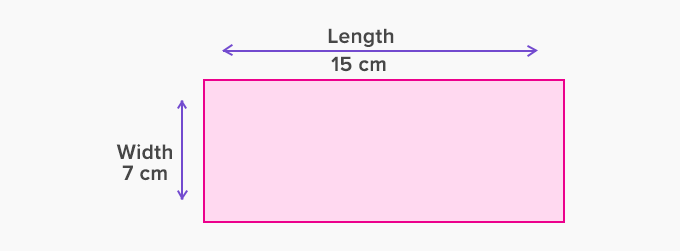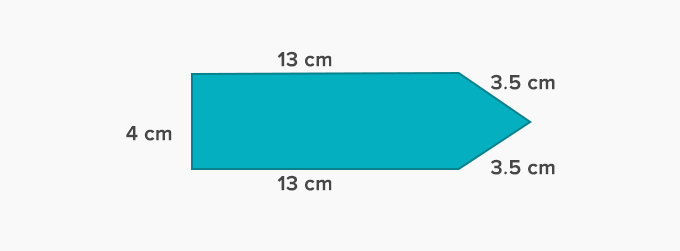Perimeter Of A Polygon - Definition with Examples

The Complete K-5 Math Learning Program Built for Your Child

• 30 Million Kids

Loved by kids and parent worldwide

• 50,000 Schools

Trusted by teachers across schools

• Comprehensive Curriculum

Aligned to Common Core

Perimeter of a Polygon

Polygon

Polygon refers to a closed 2D shape which is made up of a finite number of line segments. The number of vertices of a polygon is always equal to the number of line segments. Examples of polygons are:Perimeter of a polygon

The total distance of the outer sides of a closed figure is known as the perimeter. It is the total length of all sides of a polygon.

 Perimeter = Sum of all sides

The unit of the perimeter of any polygon will remain the same as the unit of its respective sides. If the sides are given in different units, then convert them to the same unit and then find the perimeter.

Perimeter of a regular polygon

A regular polygon has all sides equal. Therefore, we can find the perimeter of a regular polygon by repeated addition. So, we add the same length ‘n’ times if there are ‘n’ sides in the polygon.

 Perimeter of a regular polygon = (length of one side) × number of sides

Example 1: Find the perimeter of a regular hexagon with a 4 cm long side.The perimeter of a regular polygon = (length of one side) × number of sides

Perimeter of a regular hexagon = 4 cm × 6

= 24 cm

Example 2: A pentagon has all sides equal to 6 cm. What will be its perimeter?All the sides of the pentagon are equal. Therefore, it is a regular polygon.

The perimeter of a regular polygon = (length of one side) × number of sides

Perimeter of a regular pentagon = 6 cm × 5

= 30 cm

Perimeter of an irregular polygon

It is the total distance around a polygon. It can be found by adding together all the sides of the polygon.

Example 1: Work out the perimeter of the following rectangle:Perimeter = Sum of all the sides

Perimeter of rectangle = Length + Width + Length + Width

Perimeter of the rectangle = 15 cm + 7 cm + 15 cm + 7 cm = 44 cm

Hence, the formula of the perimeter of a rectangle:

 Perimeter = 2 × length + width

Example 2: Work out the perimeter of the following polygon:Perimeter = Sum of the length of all the sides

= 13 cm + 3.5 cm + 3.5 cm + 13 cm + 4 cm = 37 cm

Example 5: Area of a square is given as 100 cm2. Find its perimeter.

Area of square = 100 cm2

Formula for area of square = Side  Side

As, 100 = Side  Side

Hence, Side = 10 cm

Perimeter of a Square = Side + Side + Side + Side = 4 Sides

= 4×10=40 cm

 Fun Facts The headquarters of the US Department of Defense is called “The Pentagon” because unlike other buildings which have only four sides it has five sides.

Won Numerous Awards & Honors